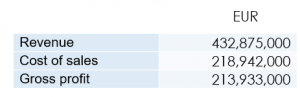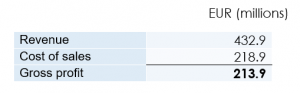# ESEF Errors and Common Pitfalls: 8 – Rounding and CalculationsThis is an addition to our series on common errors and pitfalls in ESEF filings, observed through analysis of hundreds of reports collected in our repository, at filings.xbrl.org. For the series introduction, start here.

Calculation inconsistencies are common occurrences noted in our ‘ESEF Errors and Common Pitfalls’ blog series. They occur when facts in a report do not relate to each other as expected, or in other words they do not conform to the arithmetic equations defined in a calculation tree in the taxonomy.

Calculation inconsistencies arising from facts reported with the wrong sign or from an incorrect calculation tree can — and should — be fixed, since there is a real error behind them. In this blog, we will look at another possible cause: rounding of reported figures.

Let us take this example of ‘Gross Profit’ calculated as ‘Revenue’ less the ‘Cost of Sales’. Let’s suppose that the values of these facts for a particular period are as follows:Original Values

These values satisfy the Gross Profit calculation:

432,875,000 – 218,942,000 = 213,933,000

Numeric figures in the human-readable version of reports are often scaled and rounded for better readability. Here our hypothetical company might well prefer to present these facts in millions of Euros, rounded to the nearest €100,000, as shown below.Income Statement Extract

The displayed values do not appear to satisfy the calculation relationship. The calculated value for Gross Profit would be 214.0:

432.9 – 218.9 = 214.0

This does not match the presented value of Gross Profit, which is 213.9. Although it can be surprising to see figures presented which do not add up as expected, this is purely an artefact of rounding.

Inline XBRL tags the figures exactly as they are shown in the human readable report, and so the tagged values would be the rounded values shown above, complete with the apparent calculation mismatch. XBRL processors would signal this mismatch as a calculation inconsistency, but it does not indicate a mistake in the report or its tagging.

Calculation inconsistencies are not errors, and do not invalidate the report, but they should serve as a prompt to confirm that the values are correctly reported and tagged. Preparers should review and understand all calculation inconsistencies, in order to determine whether further action is needed.

Dedicated XBRL review software can help with this process, and may also be able to help identify the different causes of calculation inconsistencies.